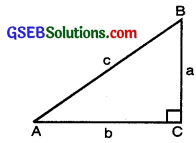# GSEB Class 10 Maths Notes Chapter 6 Triangles

This GSEB Class 10 Maths Notes Chapter 6 Triangles covers all the important topics and concepts as mentioned in the chapter.

## Triangles Class 10 GSEB Notes

We are familiar with triangles and many of their properties from earlier classes. In class IX, you have studied congruence of triangles in detail. Recall that two figures are said to be congruent, if they have the same shape and the same size. In this chapter, we shall study about those figures which have the same shape but not necessarily the same size. Two figures having the same shape (and not necessarily the same size) are called similar figures. In particular, we shall discuss the similarily of triangles and apply this knowledge in giving a simple proof of Pythagoras Theorem learnt earlier.

Similar Figures
In class IX, we have seen that all circles with the same radii are congruent, all squares with the same side lengths are congruent and all equilateral triangles with the same side lengths are congruent.
All congruent figures are similar but the similar figures need not be congruent.

Remarks
1. The polygons of the same number of sides are similar if

• their corresponding angles are equal.
• their corresponding sides are in the same ratio (or proportion).

2. Two polygons of the same number of sides are similar if:

• all the corresponding angles are equal.
• all the corresponding sides are in the same ratio (or proportion).

Similarity of Triangles
Two triangles are similar if

1. their corresponding angles are equal and
2. their corresponding sides are in the same ratio (or proportion).

If corresponding angles of two triangles are equal, then they are known as equiangular triangles. A famous Greek mathematician Thales gave an important truth relating to two equiangular triangles which is as follows: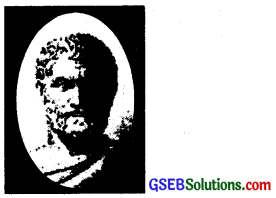Thales (640 – 546 B.C.)

The ratio of any two corresponding sides in two equiangular triangles is always the same.
It is believed that he had used a result called the Basic Proportionality Theorem (now known as the Thales Theorem) for the same.

Basic Proportionality Theorem (Thales Theorem).
Theorem:
In a triangle, a line drawn parallel to one side to intersect the Other sides in distinct points divides the two sides in the same ratio.
Given: A ∆ABC in which DE is drawn parallel to side BC intersecting sides AB and AC in points D&E respectively.

To prove: $$\frac{\mathrm{AD}}{\mathrm{DB}}=\frac{\mathrm{AE}}{\mathrm{EC}}$$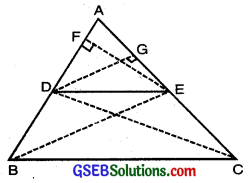Construction: Join BE, CD and through E draw EF ⊥ AB and through D draw DG ⊥ AC.

Proof: Consider ∆s ADE and DBE
ar (∆ADE) = $$\frac{1}{2}$$ AD. EF
ar(∆BDE) = $$\frac{1}{2}$$ DB. EF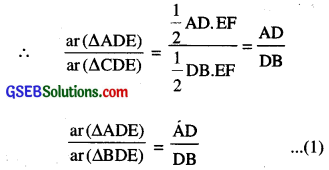Similarly by considering triangles ADE and CDE we have:
$$\frac{\operatorname{ar}(\Delta \mathrm{ADE})}{\operatorname{ar}(\Delta \mathrm{CDE})}=\frac{\mathrm{AE}}{\mathrm{EC}}$$ ……………(2)

Since two ∆s BDE and CDE are on the same base DE and between the same parallels DE and BC and so they equal in area
⇒ area (∆BDE) = area (∆CDE) ……………..(3)
From (1) and (3), we get: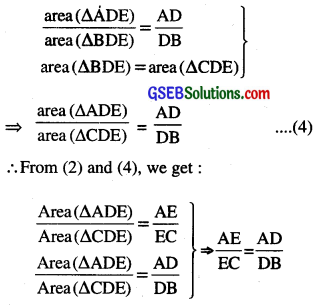Hence $$\frac{\mathrm{AD}}{\mathrm{DB}}=\frac{\mathrm{AE}}{\mathrm{EC}}$$
This proves Thales Theorem.

Corollary 1:
Thus we have proved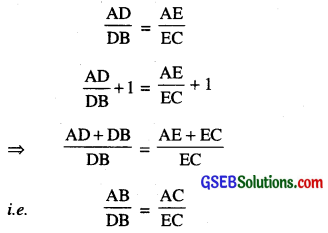Corollary 2: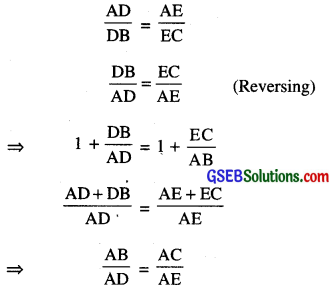Corollary 3:
$$\frac{\mathrm{DB}}{\mathrm{AD}}=\frac{\mathrm{EC}}{\mathrm{AE}}$$

Corollary 4:
$$\frac{\mathrm{DB}}{\mathrm{AB}}=\frac{\mathrm{EC}}{\mathrm{AC}}$$

Corollary 5:
$$\frac{\mathrm{AD}}{\mathrm{AB}}=\frac{\mathrm{AE}}{\mathrm{AC}}$$Theorem 2.
(Converse of Basic Proportionality Theorem)
Statement: If a line divides any two sides of a triangle in the same ratio, the line must be parallel to the third side.
Given: A ∆ABC and a line / intersecting the sides AB in D and AC in E such that
$$\frac{\mathrm{AD}}{\mathrm{DB}}=\frac{\mathrm{AE}}{\mathrm{EC}}$$

To Prove: DE ∥ BC
Proof: Let us suppose that DE is not parallel to BC. Then, through D there must be another line parallel to BC. Then, let DF ∥ BC such that E ≠ F.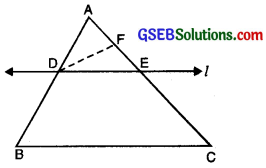DF ∥ BC (By supposition),
$$\frac{\mathrm{AD}}{\mathrm{DB}}=\frac{\mathrm{AF}}{\mathrm{FC}}$$

[Basic Proportionality Theorem] …(i)
$$\frac{\mathrm{AD}}{\mathrm{DB}}=\frac{\mathrm{AE}}{\mathrm{EC}}$$ given …….(ii)

From (i) and (ii), we get:
$$\frac{\mathrm{AF}}{\mathrm{FC}}=\frac{\mathrm{AE}}{\mathrm{EC}}$$

Adding 1 on both sides, we get: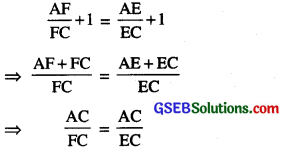⇒ $$\frac{1}{\mathrm{FC}}=\frac{1}{\mathrm{EC}}$$
FC = EC

Which shows that E and F must coincide but it contradicts our supposition that E ≠ F and DF ∥ BC
there is one and only one line, DE ∥ BC.

Theorem:
If in two triangles corresponding angles are equal i.e. the two triangles are equiangular, then triangles are similar.
Given: Two ∆s ABC and DEF in which ∠A = ∠D, ∠B = ∠E and ∠C = ∠F.
To prove: ∆ ABC ~ ∆ DEF.

Construction: Mark point M on the line DE and N on the line DF such that AB = DM and AC = DN. Join MN.
Proof: Three cases arise:
(i) AB = DE. Here M coincides with E.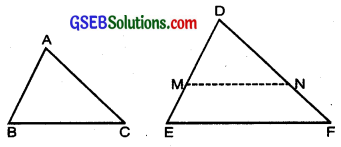In ∆s ABC and DMN,
AB = DM, AC = DN and
∠A = ZD.
∴ ∆ABC = ∆DMN [By SAS criterion]

∠B = ∠DMN [c.p.c.t]
But ∠B = ∠E (Given)
∴ ∠E = ∠DMN

Consequently. MN II EF
[If corresponding ∠s are equal, lines are parallel]
∴ $$\frac{\mathrm{DM}}{\mathrm{DE}}=\frac{\mathrm{DN}}{\mathrm{DF}}$$
[∵ If a line is drawn II to one side of a ∆, it divides the other two sides in the same ratio. (Basic Proportionality Theorem)]
$$\frac{\mathrm{AB}}{\mathrm{DE}}=\frac{\mathrm{AC}}{\mathrm{DF}}$$ …………..(1)

[ By construction AB = DM and AC = DN]
Similarly $$\frac{\mathrm{AB}}{\mathrm{DE}}=\frac{\mathrm{BC}}{\mathrm{EF}}$$ ………(2)

From (1) and (2),
$$\frac{\mathrm{AB}}{\mathrm{DE}}=\frac{\mathrm{BC}}{\mathrm{EF}}=\frac{\mathrm{AC}}{\mathrm{DF}}$$
Now ∠A = ∠D, ∠B = ∠E. ∠C = ∠F (Given)

$$\frac{\mathrm{AB}}{\mathrm{DE}}=\frac{\mathrm{BC}}{\mathrm{EF}}=\frac{\mathrm{AC}}{\mathrm{DF}}$$ (Prove above)

Hence ∆ABC ~ ∆DEF [By def. of similar ∆s]

(ii) AB > DE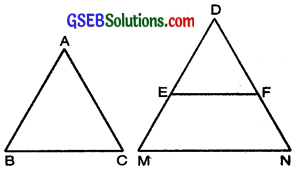Construction:
Produced DE to point M and DF to point N such that DM = AB and DN = AC.
Join MN.
Proof: In ∆ABC and ∆DMN,
AB = DM [Const.]
AC = DN [Const.]
and ∠A = ∠D [Given]
∴ ∆ABC ≅ ∆DMN [SAS congruence Axiom]
∴ ∠B = ∠DMN [CPCT]
But ∠B = ∠E = ∠DEF
⇒ ∠DMN = ∠DEF
But these are corresponding angles
∴ MN ∥ EF
$$\frac{\mathrm{DE}}{\mathrm{DM}}=\frac{\mathrm{DF}}{\mathrm{DN}}$$ [Thale’s Theorem]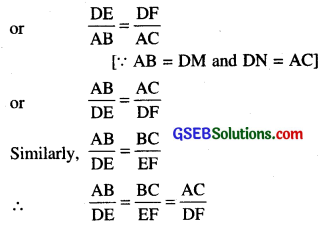By SSS similarity creterion
∆ABC ~ ∆DEF.

(iii) AB ∠ DE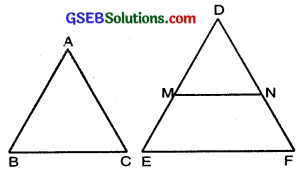Construction: Take a point M on DE and N on DF such that at AB = DM and AC = DN. Join MN.
Proof:
In ABC and ∆DMN.
AB = DM [Const.]
AC = DN [Const.]
∠A = ∠D [Given]
∴ ∆ABC s ∆DMN [SAS Congruence Axiom]

∠B = ∠DMN [CPCT]
But ∠B = ∠E = ∠DEF
∴ ∠DMN = ∠DEF
But these are corresponding angles
∴ MN ∥ EF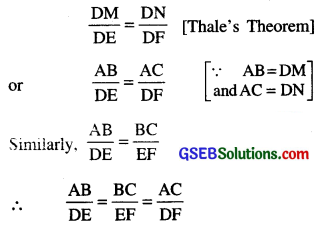By SSS congruence creterion
∆ABC ~ ∆DEF.
From above three cases, it is clear that two triangles which are equiangular are similar triangles.

Theorem:
If corresponding sides of two triangles are proportional, then they are similar.
Given: Two ∆s ABC and DEF such that
$$\frac{\mathrm{AB}}{\mathrm{DE}}=\frac{\mathrm{BC}}{\mathrm{EF}}=\frac{\mathrm{AC}}{\mathrm{DF}}$$
To prove. ∆ABC ~ ∆DEF.
Construction. Mark points M on DE such that DM = AB and N on DF such that DN = AC. Join MN.
Proof. Since $$\frac{\mathrm{AB}}{\mathrm{DE}}=\frac{\mathrm{AC}}{\mathrm{DF}}$$(Given)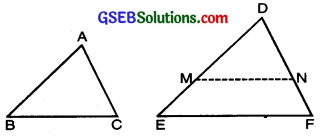But AB = DM, AC = DN [Const.]
∴ $$\frac{\mathrm{DM}}{\mathrm{DE}}=\frac{\mathrm{DN}}{\mathrm{DF}}$$
∴ MN ∥ EF
[By converse of Basic Proportionality Theorem]
⇒ ∠DMN = ∠E and ∠DNM = ∠F
[If lines area parallel, corresponding ∠s are equal]

By AAA similarity theorem,
∆ DMN ~ ∆DEF
$$\frac{\mathrm{DM}}{\mathrm{DE}}=\frac{\mathrm{MN}}{\mathrm{EF}}$$
[If ∆s are similar, the ratio of their corresponding sides is the same]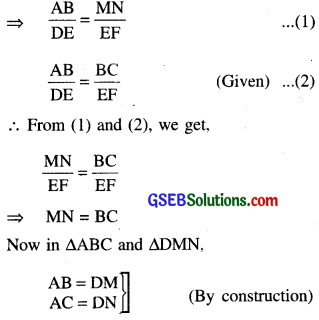and BC = MN (Proved above)
∴ ∆ABC ≅ ∆DMN [By SSS criterion of congruent ∆s]

Since ∆DMN ~ ∆DEF [Proved above]
Hence ∆ABC ~ ∆DEF.

Theorem.
If in two triangles, one pair of corresponding sides are proportional and the included angles are equal, then the two triangles are similar.
Given. Two ∆ABC and DEF such that
∠A = ∠D
and $$\frac{\mathrm{AB}}{\mathrm{DE}}=\frac{\mathrm{AC}}{\mathrm{DF}}$$

To Prove. ∆ABC ~ ∆DEF.
Construction. Mark points P and Q on DE and DF respectively such that DP = AB and DQ = AC. Join PQ.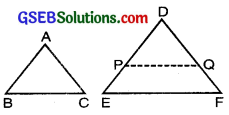Proof. In ∆ABC and DPQ, we have:
AB = DP ∠A = ∠D and AC = DQ.
∴ ∆ABC ≅ ∆DPQ [SAS Rule]

Now $$\frac{\mathrm{AB}}{\mathrm{DE}}=\frac{\mathrm{AC}}{\mathrm{DF}} \Rightarrow \frac{\mathrm{DP}}{\mathrm{DE}}=\frac{\mathrm{DQ}}{\mathrm{DF}}$$
[ AB = DP and AC = DQ]
∴ PQ ∥ EF
[by the converse of Basic Proportionality Theorem]
∴ ∠DPQ – ∠E and ∠DQP = ∠F [corresponding angles]
Hence, ∆ABC ~ ∆DEF. [∆DPQ = ∆ABC]

Result.
Two triangles are similar if

• Three angles are equal to each other (AAA)
• Corresponding sides are Proportional (SSS)
• One pair of corresponding sides are Proportional and included angles are equal (SAS)
• Two pair of corresponding angles are equal then triangles are similar (AA).Ratio of The Areas Of Two Similar Triangles
Theorem:
Prove that the ratio of the areas of two similar triangles is equal to the ratio of squares on the corresponding sides.
Given. ∆ABC ~ ∆DEF.
To Prove. $$\frac{\text { ar. }(\Delta \mathrm{ABC})}{\text { ar. }(\Delta \mathrm{DEF})}=\frac{\mathrm{BC}^{2}}{\mathrm{EF}^{2}}$$
= $$\frac{\mathrm{AB}^{2}}{\mathrm{DE}^{2}}=\frac{\mathrm{AC}^{2}}{\mathrm{DF}^{2}}$$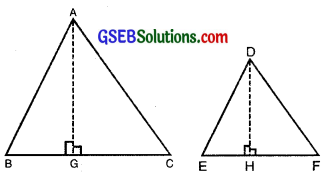Const. Draw AG ⊥ BC and DH ⊥ EF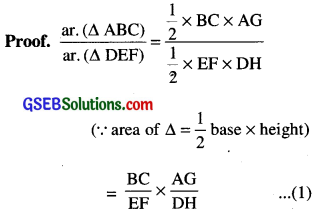Now in ∆s ABG and DEH, we have
∠B = ∠E (∵ ∆ABC ~ ∆DEF)
∠AGB = ∠DHE (each 90°)
∴ 3rd ∠BAG = 3rd ∠EDH

∆ABG – ∆DEH [AAA Similarity triangle],
∴ $$\frac{\mathrm{AB}}{\mathrm{DE}}=\frac{\mathrm{AG}}{\mathrm{DH}}$$

(∵ If ∆s are similar, the ratio of their corresponding sides is same)
AB BC
But $$\frac{\mathrm{AB}}{\mathrm{DE}}=\frac{\mathrm{BC}}{\mathrm{EF}}$$ [∵ ∆ABC ~ ∆DEF] (Given)
∴ $$\frac{\mathrm{AG}}{\mathrm{DH}}=\frac{\mathrm{BC}}{\mathrm{EF}}$$ ……..(2)

From (1) and (2)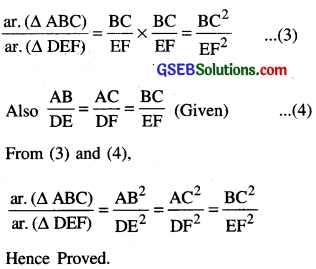Pythagoras Theorem
Theorem.
In a right triangle, the square of the hypotenuse, is equal to the sum of the squares of the other two sides.
Given: A right triangle ABC, right angled at B.
To prove: AC2 = AB2 + BC2.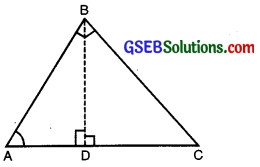Construction: Draw BD ⊥ AC.
Proof: In ∆ADB and ∆ABC, we have
∠ADB = ∠ABC (Each = 90°)
∠A = ∠A (Common)
∴ ∆ABD ~ ∆ABC
(By AA criterion of similarity)
∴ $$\frac{\mathrm{AD}}{\mathrm{AB}}=\frac{\mathrm{AB}}{\mathrm{AC}}$$
[∵ Corresponding sides of similar triangles are proportional]
⇒ AB2 = AD × AC …(1)

Similarly, ∆BDC ~ ∆ABC
∴$$\frac{\mathrm{BC}}{\mathrm{AC}}=\frac{\dot{\mathrm{DC}}}{\mathrm{BC}}$$
⇒ BC2 = DC × AC …(2)
Adding (1) and (2), we get,
AB2 + BC2 = AD × AC + DC × AC
= (AD + DC) × AC
= AC × AC = AC2
Hence AC2 = AB2 + BC2.

Converse of the Pythagoras Theorem
Statement: In a triangle, if the square of one side is equal to the sum of the squares of the other two sides, then the angle opposite to the first side is a right angle.
Given: A triangle ABC such that AB2 + BC2 = AC2
To prove: ∠ABC = 90°
Construction: Construct a right triangle PQR, right angled at Q, such that PQ = AB and QR = BC.
Proof: ∵ In APQR, ZPQR = 90°
∴ PQ2 + QR2 = PR2 (Pythagoras Theorem)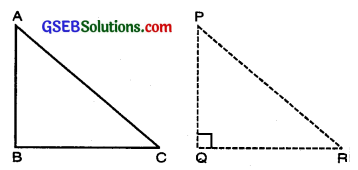But PQ = AB and QR = BC (By construction)

∴ AB2 + BC2 = PR2 …(1) [By Pythagoras Theorem]
But AB2 + BC2 = AC2 …(2) (Given)
From (1) and (2), we get
PR2 = AC2
⇒ PR = AC
In ∆ABC and ∆PQR, we have AB = PQ, BC = QR and AC = PR
∴ ∆ABC ≅ ∆PQR
[By SSS congruence criterion]

⇒ ∠ABC = ∠PQR [c.p.c.t]
But ∠PQR = 90° (By construction)
Hence ∠ABC = 90°.
Some result

Pythagoras Triplet
If c is the length of the hypotenuse, and a and b are the lengths of other two sides, the Pythagoras theorem is
c2 = a2 + b2

A set of Pythagoras triplet is a set of three natural numbers which satisfy the relation.
c2 = a2 + b2